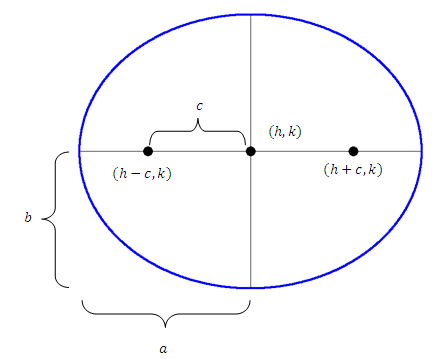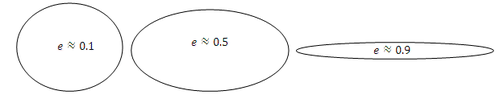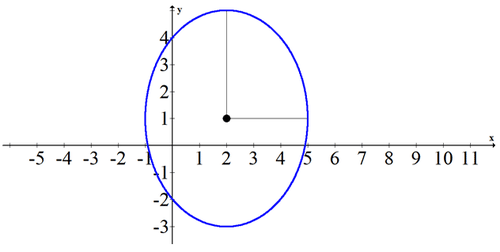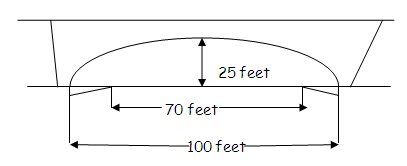# 9.4 Ellipses

$$\newcommand{\vecs}{\overset { \rightharpoonup} {\mathbf{#1}} }$$ $$\newcommand{\vecd}{\overset{-\!-\!\rightharpoonup}{\vphantom{a}\smash {#1}}}$$$$\newcommand{\id}{\mathrm{id}}$$ $$\newcommand{\Span}{\mathrm{span}}$$ $$\newcommand{\kernel}{\mathrm{null}\,}$$ $$\newcommand{\range}{\mathrm{range}\,}$$ $$\newcommand{\RealPart}{\mathrm{Re}}$$ $$\newcommand{\ImaginaryPart}{\mathrm{Im}}$$ $$\newcommand{\Argument}{\mathrm{Arg}}$$ $$\newcommand{\norm}{\| #1 \|}$$ $$\newcommand{\inner}{\langle #1, #2 \rangle}$$ $$\newcommand{\Span}{\mathrm{span}}$$ $$\newcommand{\id}{\mathrm{id}}$$ $$\newcommand{\Span}{\mathrm{span}}$$ $$\newcommand{\kernel}{\mathrm{null}\,}$$ $$\newcommand{\range}{\mathrm{range}\,}$$ $$\newcommand{\RealPart}{\mathrm{Re}}$$ $$\newcommand{\ImaginaryPart}{\mathrm{Im}}$$ $$\newcommand{\Argument}{\mathrm{Arg}}$$ $$\newcommand{\norm}{\| #1 \|}$$ $$\newcommand{\inner}{\langle #1, #2 \rangle}$$ $$\newcommand{\Span}{\mathrm{span}}$$$$\newcommand{\AA}{\unicode[.8,0]{x212B}}$$

An ellipse is commonly known as an oval. Ellipses are just as common as parabolas in the real world with their own uses. Rooms that have elliptical shaped ceilings are called whisper rooms because if you stand at one focus point and whisper, someone standing at the other focus point will be able to hear you.

Ellipses look similar to circles, but there are a few key differences between these shapes. Ellipses have both an $$x$$ -radius and a $$y$$ -radius while circles have only one radius. Another difference between circles and ellipses is that an ellipse is defined as the collection of points that are a set distance from two focus points while circles are defined as the collection of points that are a set distance from one center point. A third difference between ellipses and circles is that not all ellipses are similar to each other while all circles are similar to each other. Some ellipses are narrow and some are almost circular. How do you measure how strangely shaped an ellipse is?

## Graphing Ellipses

An ellipse has two foci. For every point on the ellipse, the sum of the distances to each foci is constant. This is what defines an ellipse. Another way of thinking about the definition of an ellipse is to allocate a set amount of string and fix the two ends of the string so that there is some slack between them. Then use a pencil to pull the string taught and trace the curve all the way around both fixed points. You will trace an ellipse and the fixed end points of the string will be the foci. Foci is the plural form of focus. In the picture below, $$(h, k)$$ is the center of the ellipse and the other two marked points are the foci.The major axis is the longest distance from end to end of an ellipse and is twice as long as the semi-major axis. The semi-major axis is the distance from the center of the ellipse to the furthest point on the ellipse and the semi-minor axis is the distance from the center to the edge of the ellipse on the axis that is perpendicular to the semi-major axis.

The general equation for an ellipse is:

$$\frac{(x-h)^{2}}{a^{2}}+\frac{(y-k)^{2}}{b^{2}}=1$$

In this case the major axis is horizontal because $$a,$$ the $$x$$ -radius, is larger. If the $$y$$ -radius were larger, then $$a$$ and $$b$$ would reverse. In other words, the coefficient $$a$$ always comes from the length of the semi major axis (the longer one) and the coefficient $$b$$ always comes from the length of the semi minor axis (the shorter one).

In order to find the locations of the two foci, you will need to find the focal radius represented as $$c$$ using the following relationship:

$$a^{2}-b^{2}=c^{2}$$

Once you have the focal radius, measure from the center along the major axis to locate the foci. The general shape of an ellipse is measured using eccentricity. Eccentricity is a measure of how oval or how circular the shape is. Ellipses can have an eccentricity between 0 and 1 where a number close to 0 is extremely circular and a number close to 1 is less circular. Eccentricity is calculated by:

$$e=\frac{c}{a}$$

Ellipses also have two directrix lines that correspond to each focus but on the outside of the ellipse. The distance from the center of the ellipse to each directrix line is $$\frac{a^{2}}{c}$$

## Examples

##### Example 1

Earlier, you were asked how you measure how strangely shaped an ellipse is. Ellipses are measured using their eccentricity. Here are three ellipses with estimated eccentricity for you to compare.Eccentricity is the ratio of the focal radius to the semi major axis: $$e=\frac{c}{a}$$

##### Example 2

Find the vertices (endpoints of the major axis), foci and eccentricity of the following ellipse.

$$\frac{(x-2)^{2}}{4}+\frac{(y+1)^{2}}{16}=1$$

The center of the ellipse is at (2,-1) . The major axis is vertical which means the semi major axis is $$a=4$$. The vertices are (2,3) and (2,-5)

\begin{aligned} 16^{2}-4^{2} &=c^{2} \\ 4 \sqrt{15}=\sqrt{240} &=c \end{aligned}

Thus the foci are $$(2,-1+4 \sqrt{15})$$ and $$(2,-1-4 \sqrt{15})$$

##### Example 3

Sketch the following ellipse.

$$\frac{(y-1)^{2}}{16}+\frac{(x-2)^{2}}{9}=1$$

Plotting the foci are usually important, but in this case the question simply asks you to sketch the ellipse. All you need is the center, $$x$$ -radius and $$y$$ -radius.##### Example 4

Put the following conic into graphing form.

$$25 x^{2}-150 x+36 y^{2}+72 y-639=0$$

\begin{aligned} 25 x^{2}-150 x+36 y^{2}+72 y-639 &=0 \\ 25\left(x^{2}-6 x\right)+36\left(y^{2}+2 y\right) &=639 \\ 25\left(x^{2}-6 x+9\right)+36\left(y^{2}+2 y+1\right) &=639+225+36 \\ 25(x-3)^{2}+36(y+1)^{2} &=900 \\ \frac{25(x-3)^{2}}{900}+\frac{36(y+1)^{2}}{900} &=\frac{900}{900} \\ \frac{(x-3)^{2}}{36}+\frac{(y+1)^{2}}{25}=1 & \end{aligned}

##### Example 5

Put the following conic into graphing form.

$$9 x^{2}-9 x+4 y^{2}+12 y+\frac{9}{4}=-8$$

$$9 x^{2}-9 x+4 y^{2}+12 y+\frac{9}{4}=-8$$

$$9 x^{2}-9 x+\frac{9}{4}+4 y^{2}+12 y=-8$$

$$9\left(x^{2}-x-\frac{1}{4}\right)+4\left(y^{2}+3 y\right)=-8$$

$$9\left(x-\frac{1}{2}\right)^{2}+4\left(y^{2}+3 y+\frac{9}{4}\right)=-8+4 \cdot \frac{9}{4}$$

$$9\left(x-\frac{1}{2}\right)^{2}+4\left(y+\frac{3}{2}\right)^{2}=1$$

$$\frac{\left(x-\frac{1}{2}\right)^{2}}{\frac{1}{9}}+\frac{\left(y+\frac{3}{2}\right)^{2}}{\frac{1}{4}}=1$$

##### Review

Find the vertices, foci, and eccentricity for each of the following ellipses.

1. $$\frac{(x-1)^{2}}{4}+\frac{(y+5)^{2}}{16}=1$$

2. $$\frac{(x+1)^{2}}{9}+\frac{(y+2)^{2}}{4}=1$$

3. $$(x-2)^{2}+\frac{(y-1)^{2}}{4}=1$$

Now sketch each of the following ellipses (note that they are the same as the ellipses in #1-#3).

4. $$\frac{(x-1)^{2}}{4}+\frac{(y+5)^{2}}{16}=1$$

5. $$\frac{(x+1)^{2}}{9}+\frac{(y+2)^{2}}{4}=1$$

6. $$(x-2)^{2}+\frac{(y-1)^{2}}{4}=1$$

Put each of the following equations into graphing form.

7. $$x^{2}+2 x+4 y^{2}+56 y+197=16$$

8. $$x^{2}-8 x+9 y^{2}+18 y+25=9$$

9. $$9 x^{2}-36 x+4 y^{2}+16 y+52=36$$

Find the equation for each ellipse based on the description.

10. An ellipse with vertices (4,-2) and (4,8) and minor axis of length $$6 .$$

11. An ellipse with minor axis from (4,-1) to (4,3) and major axis of length 12 .

12. An ellipse with minor axis from (-2,1) to (-2,7) and one focus at (2,4) .

13. An ellipse with one vertex at $$(6,-15),$$ and foci at (6,10) and (6,-14)

A bridge over a roadway is to be built with its bottom the shape of a semi-ellipse 100 feet wide and 25 feet high at the center. The roadway is to be 70 feet wide.14. Find one possible equation of the ellipse that models the bottom of the bridge.

15. What is the clearance between the roadway and the overpass at the edge of the roadway?

...

This page titled 9.4 Ellipses is shared under a CK-12 license and was authored, remixed, and/or curated by CK-12 Foundation via source content that was edited to the style and standards of the LibreTexts platform; a detailed edit history is available upon request.